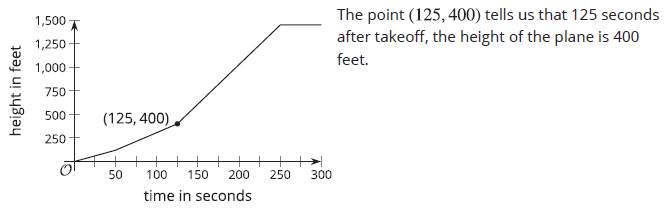# A.4.1 Lesson Summary

A relationship between two quantities is a function if there is exactly one output for each input. We call the input the independent variable and the output the dependent variable. Let’s look at the relationship between the amount of time since a plane takes off, in seconds, and the plane’s height above the ground, in feet. • These two quantities form a function if time is the independent variable (the input) and height is the dependent variable (the output). This is because at any amount of time since takeoff, the plane could only be at one height above the ground.                 For example, 50 seconds after takeoff, the plane might have a height of 180 feet. At that moment, it cannot be simultaneously 180 feet and 95 feet above the ground.                 For any input, there is only one possible output, so the height of the plane is a function of the time since takeoff. • The two quantities do not form a function, however, if we consider height as the input and time as the output. This is because the plane can be at the same height for multiple lengths of times since takeoff.               For instance, when the plane is 1,500 feet above ground, it is possible that 300 seconds have passed. It is also possible that 425 seconds, 275 seconds, or some other amounts of time have passed.                 For any input, there are multiple possible outputs, so the time since takeoff is not a function of the height of the plane. Functions can be represented in many ways—with a verbal description, a table of values, a graph, an expression or an equation, or a set of ordered pairs. When a function is represented with a graph, each point on the graph is a specific pair of input and output. Here is a graph that shows the height of a plane as a function of time since takeoff.